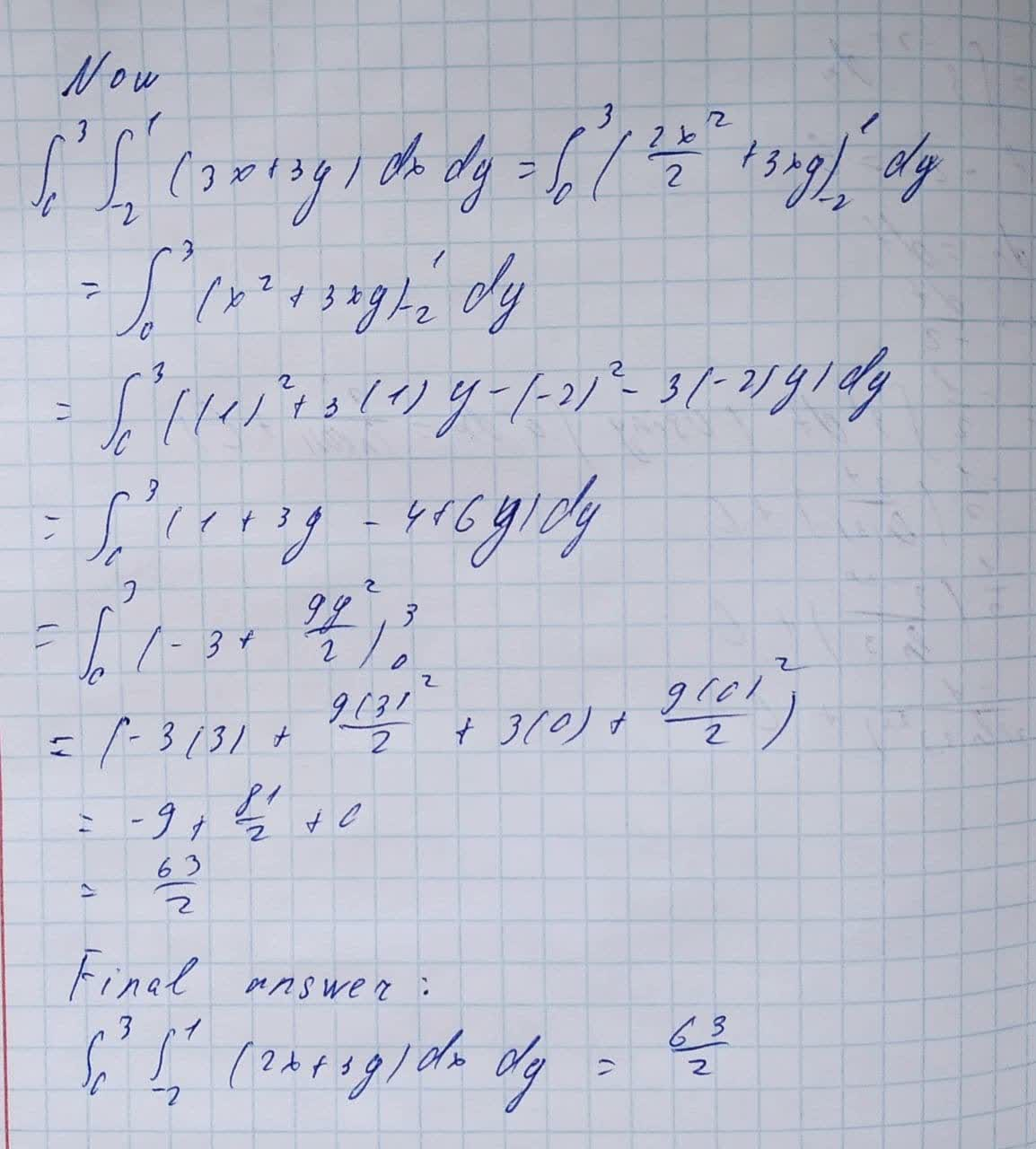Question# Evaluate the following iterated integrals. \int_{0}^{3}\int_{-2}^{1}(2x+3y)dx dy

Applications of integrals
ANSWEREDEvaluate the following iterated integrals.
$$\displaystyle{\int_{{{0}}}^{{{3}}}}{\int_{{-{2}}}^{{{1}}}}{\left({2}{x}+{3}{y}\right)}{\left.{d}{x}\right.}{\left.{d}{y}\right.}$$